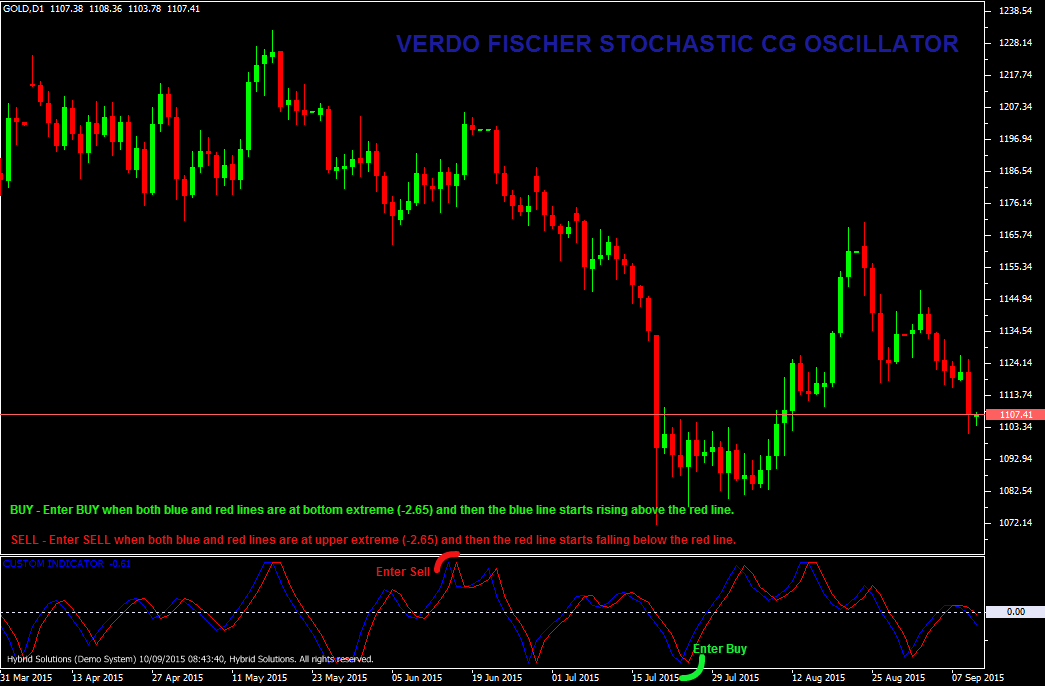# Fischer Stochastic CG Oscillator

Free

Developed By: NeeduVerdeSolution

In stock

Fischer Stochastic CG (Center of Gravity) oscillator is a VertexFX Indicator created from the CG (Center of Gravity) Oscillator in three steps. In the first step we calculate the CG value, then we apply stochastic filter to the CG oscillator, and finally we apply a Fischer transform to this result. This indicator is useful to detect balance of the market, and when market deviates from this balance it can be an important turning point of the market.

SKU: 678 Categories: ,Fischer Stochastic CG (Center of Gravity) oscillator is a VertexFX Indicator created from the CG (Center of Gravity) Oscillator in three steps. In the first step we calculate the CG value, then we apply stochastic filter to the CG oscillator, and finally we apply a Fischer transform to this result. This indicator is useful to detect balance of the market, and when market deviates from this balance it can be an important turning point of the market.

Fischer Stochastic CG (Center of Gravity) Oscillator comprises of two components, Fischer Stochastic CG indicator (Blue) and the Trigger (Red). A zero counter value for CG is established by adding half observation window (Length) plus 1. Since the CG (Center of Gravity) is smoothed, an effective crossover signal is produced simply by delaying the CG (Center of Gravity) by one bar. Now, this value of CG (Center of Gravity) is normalized based on the highest and lowest values over the length bars, and then weighted using WMA to generate the Stochastic CG.

BUY – Place Buy position when both blue and red lines are at bottom extreme (-2.65) and blue line starts rising above red line.

SELL – Place Sell when both blue and red lines are at upper extreme (+2.65) and red line starts falling below the red line.